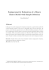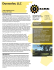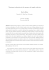# Recent Two-Stage Sample Selection Procedures with an

## Transcription

Recent Two-Stage Sample Selection Procedures with an
```Recent Two-Stage Sample Selection Procedures with an
Application to the Gender Wage Gap∗
Louis N. Christofides
Department of Economics
University of Cyprus
Kallipoeos 75, 1678 Nicosia, Cyprus
Qi Li
Department of Economics
Texas A&M University
College Station, TX 77843, U.S.A.
Zhenjuan Liu
Department of Economics
University of Guelph
Insik Min
Department of Economics
Texas A&M University
College Station, TX 77843, U.S.A.
Abstract
Recently-developed two-stage estimation methods of sample selection models are
used, in the context of data from the 1989 Labor Market Activity Survey, to examine
labor supply decisions and wage outcomes for employed men and women. Recent
hypothesis test procedures are used to test for no sample selection, and to test for
a parametric against a semiparametric selection-correction procedure. We conclude
that selection is indeed an issue for the sample at hand and that the semiparametric
specification is appropriate. We also present the standard decomposition of the gender
wage gap into its explained and unexplained portions.
JLE classification: C14, C24, C51, J22, J31.
Keywords: Two-stage semiparametric estimation, selection, labor supply, wage outcomes.
∗
We thank two referees, an associate editor, and Jeff Wooldridge for insightful comments that greatly
improved the paper. We would also like to thank R. Swidinsky, B. Wandschneider and D. Wang for their
Enterprise Research Center, and the Bush Program in Economics of Public Policy, Texas A&M University.
Christofides thanks the University of Guelph, where he is Adjunct Professor.
1
Introduction
Empirical work in many areas of economics involves working with sub-samples which are
drawn from random samples of the population according to specified criteria. For instance,
studies of the wage determination process typically involve sub-samples of individuals who
are employed, work positive hours, and have known wages; the researcher may be interested
in the extent to which socio-economic characteristics may affect wages. The extent to which
results based on selected samples can be meaningful has been discussed at least as far back
as Gronau (1974) and Lewis (1974). While a number of approaches have been considered,
Heckman (1976,1979) suggested the most widely-used procedure: A process describing the
employment outcome is implemented and information from this is used, in a second stage,
to obtain consistent estimates of the relevant parameters. It is well-known that this twostage procedure can be subject to data-driven problems. These include lack of identification
and results which are sensitive to specification. For a careful discussion of this problem in
a particular empirical context, see Baker et al (1995). For more recent developments, see
Heckman (1990), Manski (1989, 1990), Newey et al (1990), and Vella (1993,1998),
Vella (1992) proposes a test for sample selection bias using a Type-3 Tobit residual
as a generated regressor. Using a similar idea, Wooldridge (1994) proposes a two-stage
estimator which is simple to use and is more robust than Heckman’s procedure. Wooldridge
(1995) further considers the sample selection bias problem with panel data. Wooldridge
(1994) also shows that his method can be generalized to allow for non-normality in the
error distributions, a possibility that would render application of the Heckman (1976,1979)
technique inappropriate. In this case, the model becomes a semiparametric partially linear
model with generated regressors entering the model nonparametrically. Li and Wooldridge
√
(2002) derive a n-consistent estimator for this model. Alternative semiparametric, twostage, methods that do not require knowledge of error distributions are proposed by various
authors, see Chen (1997), Honore, Kyriazidou and Udry (1997), and Lee (1994), among
others. Recently developed consistent model specification tests (e.g., Zheng (1996), and Li
and Wang (1998) among others) provide the background for hypothesis tests of selection bias
and, in the event that selection bias is accepted, one can further test for a parametric selection
1
null model versus a semiparametric alternative. Thus a coherent, two-stage, approach which
overcomes some difficulties that may arise in the Probit-OLS sequence and which tests
down from the general (selection versus no selection) to the particular (parametric versus
semiparametric correction) is now available.
In this paper, we make extensive and integrated use of these recent developments by
considering the problem of estimating labour market involvement and wage equations from
samples of employed men and women. Using selection-corrected wage equations, we also
consider the gender wage gap and its traditional decomposition into portions explainable by
characteristics and possible discrimination. We rely on the very large samples that can be
drawn from the 1989 Labour Market Activity Survey for Canada, a source for earlier studies
of the wage determination process that use the Heckman (1976,1979) approach.
In section 2, we briefly discuss some recent semiparametric estimation methods for the
Type-3 Tobit model. These will provide the econometric background required in the applied
analysis. In section 3, we describe the data. In section 4, we provide labour supply and wage
equations based on the alternative estimators and conduct the hypothesis tests required to
select the appropriate model. We also report the results of decomposing observed male-female
wage differentials into portions attributable to characteristics and possible discrimination.
Concluding comments appear in section 5.
2
Econometric Preliminaries
Consider the Type-3 Tobit model defined by the latent variables
y1∗ = x1 β1 + u1 ,
(1)
y2∗ = x2 β2 + u2 ,
(2)
where the first equation is the selection equation and the second equation is the main equation
of interest. The dependent variable y2∗ can only be observed when the selection variable y1∗
is positive. Thus we observe y1 and y2 which satisfy
y1 = max{y1∗ , 0},
(3)
y2 = y2∗ 1{y1 >0} ,
(4)
2
where 1{A} represents the indicator function of the event A, y1 and y2 are the observable
dependent variables, x1 and x2 are row vectors of exogenous variables with dimension p1 and
p2 respectively, and β1 and β2 are conformable column vectors of unknown parameters. In
the empirical application considered in section 4, y1 is the working hours of an individual
and y2 is the logarithm of the hourly wage rate.
Under the selection rule described by Eq. (3) and Eq. (4), we have
E(y2∗ |x1 , x2 , y1∗ > 0) = x2 β2 + E(u2 |u1 > −x1 β1 , x1 , x2 ).
(5)
Hence, the least squares method of regressing y2 on x2 is an inconsistent estimator of β2 if
the second term on the right-hand-side of Eq. (5) is non-zero. Under the joint normality assumption of (u1 , u2 ), Heckman (1976, 1979) proposes a simple, two-stage, method to estimate
Type-2 or Type-3 Tobit models. Heckman’s suggestion was to restore a zero conditional mean
in Eq. (4), by including an estimate of the selection bias term, E(u2 |u1 > −x1 β1 , x1 , x2 ).
Under normality, this term is proportional to the inverse Mills ratio (we use λ to denote it),
and depends only on unknown parameters of Eq. (1) which can be estimated by Probit or
Tobit maximum likelihood.
Vella (1992, 1998) and Wooldridge (1994) suggest alternative two-stage estimation methods that may have better finite sample properties. Under the assumption that (x1 , x2 ) are independent of (u1 , u2 ), Vella and Wooldridge note that E(u2 |x, u1 , y1 > 0) = E(u2 |u1 , y1 > 0).
If one further assumes that E(u2 |u1 ) = γ1 u1 , then the selection bias correction term is γ1 u1 .
One can estimate u1 by uˆ1 = y1 − x1 βˆ1 , where βˆ1 is the Tobit estimator of β1 . Thus one
can use u1 , rather than Heckman’s (1979) inverse Mills ratio, as an additional variable in
the conditional expectation. The advantage is that, even when x2 and the inverse Mills
ratio are near collinearity, u1 has more variation than x2 thereby rendering Vella-Wooldridge
estimator more stable and therefore more efficient, see Wooldridge (2002, p.573) for a more
detailed discussion on this.
One can also estimate (3) and (4) simultaneously with the maximum likelihood method
by assuming the joint normality of (u1 , u2 ). Compared with the maximum likelihood method,
the Vella-Wooldridge two-step method has at least three advantages: (i) it is computationally
less costly, (ii) it does not require the joint normality of (u1 , u2 ), only assuming the normality
3
of u1 and that E(u2 |u1 ) is linear in u1 , and (iii) it is more robust to near collinearity in data.
As noted by Wooldridge (1994), a further advantage of the Vella-Wooldridge approach
is that the assumption of normality can be easily relaxed. There is no need to assume the
joint distribution of (u1 , u2 ) to be known, or to assume that E(u2 |u1 ) = γ1 u1 . When the
joint distribution of (u1 , u2 ) is unknown, one has E(u2 |u1 ) = g(u1 ), where g(.) is an unknown
function. In this case one can easily show that E(y2i |xi , u1i ) = x2i β2 + g(u1i ). Thus we have
y2i = x2i β2 + g(u1i ) + v2i ,
(6)
where v2i satisfies E(v2i |u1i , y1i > 0) = 0.
Following Robinson (1988) and using the data with y1i > 0, we get from (6)
y2i − E(y2i |u1i ) = [x2i − E(x2 |u1i )]β2 + v2i .
(7)
Li and Wooldridge (2002) suggest a two-step method to estimate β2 . (i) Estimate u1i by
uˆ1i = y1i − x1i βˆ1 , where βˆ1 is a first-stage estimator of β1 , say Powell’s (1984) censored least
absolute deviation (CLAD) estimator defined by
n
X
1
βˆ1 = argminβ1
|y1i − max{0, x1i β1 }|,
n i=1
(8)
1
then (ii) use {y2i , x2i , uˆ1i }ni=1
to obtain nonparametric kernel estimates of E(y2i |u1i ) and
E(x2i |u1i ), and finally (iii) apply a least squares method to estimate β2 based on (7) (e.g.,
√
Robinson (1988)). Li and Wooldridge (2002) also establish the n-normality of their estimator for β2 (denote it by βˆ2,LW ).
A number of other authors have also suggested semiparametric estimation of Type-3
Tobit models that do not require knowledge of the joint distribution of (u1 , u2 ), see Chen
(1997), Honore et al (1997), and Lee (1994), among others. Below, we briefly discuss some
of the estimators proposed by these authors.
Chen (1997) observes that, under the condition that (u1 , u2 ) is independent of (x1 , x2 ),
E(y2 |x1 , x2 , u1 > 0, x1 β1 > 0, y1 > 0) = E(y2 |u1 > 0, x) = x2 β2 + α0 ,
4
(9)
where α0 is a constant, but α0 is not the intercept of the original model because an intercept
is not identified without further assumptions. Based on (9) Chen suggests a simple leastsquares procedure applied to a trimmed subsample to estimate β2 by
n
X
1
βˆ2,Chen = argminβ2 ,α
1
(y2i − x2i β2 − α)2 ,
ˆ
ˆ
n i=1 {y1i −x1i β1 >0,x1i β1 >0}
(10)
√
where βˆ1 is a n-consistent estimator of β1 in a first step, say the estimator proposed by
Honore and Powell (1994), or the CLAD estimator of Powell (1984). As discussed in Chen,
one problem with the estimator given by (10) is that it may trim out too many observations
and hence lead to inefficient estimation. Chen (1997) further suggests an alternative estimator that trims much less data points in finite sample applications (see Eq. (11) of Chen
(1997) for details).
Honore, Kyriazidou and Udry’s (1997, hereafter HKU) consider an alternative approach.
To relax the normality assumption of Heckman, HKU (1997) consider the case where the
underlying errors are symmetrically distributed conditional on the regressors, with arbitrary
heteroskedasticity permitted. The effect of sample selection in this case is that the errors
are no longer symmetrically distributed conditional on the sample selection. HKU (1997)
note that if one estimated β2 using observations for which −x1 β1 < u1 < x1 β1 (equivalent to
0 < y1 < 2x1 β1 ), u2 is symmetrically distributed around 0 under this conditioning. Hence,
the following least absolute deviations estimator consistently estimates β2 :
n
X
1
1
βˆ2,HKU = argminβ2
ˆ |y2i − x2i β2 |,
n i=1 {0<y1i <2x1i β1 }
(11)
√
where βˆ1 is a first stage n-consistent estimator of β1 , say Powell’s (1984) censored least
√
absolute deviations estimator defined above. HKU (1997) also establish the n-normality
of their proposed estimator βˆ2,HKU .
Under the assumption of independence between errors and regressors, Lee (1994. Eq.
2.12) shows that
y2i − E(y2 |u1 > −x1i β1 , x1 β > x1i β1 ) = [x2i − E(x2 |x1 β1 > x1i β1 )]β2 + 2i ,
(12)
where 2i satisfies E(2i |u1 > −x1i β1 , x1 β > x1i β1 ) = 0. Lee (1994) suggests to first replace
the conditional expectations in (12) by kernel estimators (also β1 needs to be replaced by a
5
first stage estimator, say βˆ1 given in Powell (1984)), and then apply a least squares procedure
to estimate β2 (denote it by βˆ2,Lee ). Lee (1994) establishes the asymptotic normal distribution
of βˆ2,Lee .
Chen’s (1997) and HKU’s (1997) methods do not require nonparametric estimation techniques, while Li and Wooldridge (2002) and Lee (1994) use the nonparametric kernel estimation method. It is known that nonparametric kernel estimation may be sensitive to the
choice of smoothing parameter. However, the Monte Carlo simulations in Lee (1994) and
Sheu (2000) suggest that Lee’s and Li and Wooldridge’s estimators are not very sensitive to
smoothing parameter choices. In particular for the Li-Wooldridge method, Sheu (2000) uses
two different methods to select the smoothing parameter. One is by the least squares cross−1/5
validation method, the other is an ad-hoc rule with h = cˆ
u1,sd n1
standard deviation of
, where uˆ1,sd is the sample
1
{ˆ
u1i }ni=1
and c is a constant between 0.8 to 1.2. Sheu (2000) finds that
the estimated mean squared errors of βˆ2 are quite similar to the different choices of h. The
reason is that the semiparametric estimator β2 depends on the average of the nonparametric
estimators, and an average nonparametric estimator is less sensitive to different values of the
smoothing parameters than, say, a point-wise nonparametric kernel estimator. Therefore, in
this paper we will use the simple ad-hoc method to select the smoothing parameters (with
the constant c = 1).
We also consider a semiparametric Type-2 Tobit model where we use Ichimura’s (1993)
semiparametric nonlinear least squares (SNLS) method to estimate β1 based on a single
index model with the binary labour force participation data. Using data with y1i > 0, the
corresponding semiparametric wage equation is a partially linear single index model (e.g.
Ichimura and Lee (1991))
y2i = x2i β2 + θ(x1i β1 ) + η2i ,
(13)
where θ(x1i β1 ) = E(u2 |u1 > −x1i β) is of unknown functional form, and η2i satisfies the
condition E(η2i |xi ) = 0. Ichimura and Lee (1991) propose a semiparametric NLS method to
estimate model (13) and they have established the asymptotic distribution of their proposed
estimator.
In this paper, we consider four parametric estimation methods: (P1) the Vella-Wooldridge
6
parametric approach (denote it by VW), (P2) Heckman’s two-stage method, (P3) OLS estimation, and (P4) joint maximum likelihood estimation based on the joint normality of
(u1 , u2 ). We consider five semiparametric estimation methods: (S1) The semiparametric estimator by Chen (1997), (S2) the semiparametric estimator by HKU (1997), (S3) the semiparametric estimator by Lee (1994), (S4) the semiparametric estimator by Li and Wooldridge
(2002) (denote it by LW), and (S5) the semiparametric Type-2 Tobit estimator based on
Ichimura (1993) and Ichimura and Lee (1991). Note that HKU require that u2 have a
(conditional) symmetric distribution, but they do not require (u1 , u2 ) to be independent of
(x1 , x2 ); on the other hand, Chen, Ichimura, Ichimura and Lee, Lee, and Li and Wooldridge
assume that (u1 , u2 ) is independent of (x1 , x2 ), but u2 need not be symmetrically distributed.
The symmetry condition is neither weaker, nor stronger than the independence condition.
Turning to tests of selection bias, we focus on testing for no selection bias, or a parametric selection bias as described in Vella (1992) and Wooldridge (1994), against general
semiparametric selection bias as described in Li and Wooldridge (2002). Denote the null
hypothesis of no selection bias as H0a . If H0a is rejected, it is necessary to test whether a
def
parametric selection model is adequate, that is, whether H0b : E(u2 |u1 ) = g(u1 ) = u1 γ almost
everywhere. If the errors are normally distributed, then g(u1 ) = u1 γ and one can test for no
selection bias by testing whether γ = 0. However, when g(u1 ) 6= u1 γ, the parametric test for
no selection bias based on testing γ = 0 can give misleading results. Both types of mistakes
can occur: When H0a is true, this test may reject the null hypothesis when g(u1 ) 6= u1 γ.
When H0a is false, the parametric test can have no power, even as the sample size tends to
infinity, because it is not a consistent test.
The test statistic below is robust to different distributional assumptions regarding (u1 , u2 ).
That is, no matter what the joint distribution of (u1 , u2 ), if there is a selection bias the probability of detecting it will converge to one as the sample size goes to infinity. The null
hypothesis of no selection bias (H0a ) can be stated as E(u2 |u1 ) = 0. The alternative hypothesis (H1a ) can be stated as E(u2 |u1 ) ≡ g(u1 ) 6= 0. If H0a is true, then the OLS regression
of the observed y2 on x2 gives a consistent estimator for β2 under H a (denote it by βˆ2,ols ),
0
and the least squares residual: uˆ2i = y2i − x2i βˆ2,ols is a consistent estimator of u2i (under
H0a ). Similar to the test statistic for model specification proposed by Li and Wang (1998)
7
and Zheng (1996), a test statistic for H0a is given by
Ina =
n1
n1
X
1 X
uˆ1i − uˆ1j
).
u
ˆ
u
ˆ
K(
2i
2j
n21 h i=1 j6=i,j=1
h
(14)
where n1 denotes the observed sample of y2 and uˆ1i = y1i − x1i βˆ1 .
We give some regularity conditions under which one can derive the asymptotic distribution of Ina , as well as another test Inb defined below.
(C1) (y2i , xi , u1i , u2i ) are i.i.d. as (y2 , x, u1 , u2 ). x, u1 and u2 all have finite fourth moments. ∂g(u1 )/∂u1 , ∂ 2 g(u1 )/∂u21 are continuous in u1 and dominated by a function (say
M (u1 )) with finite second moment. βˆ1 − β1 = Op (n−1/2 ).
(C2) The kernel function K(.) is bounded, symmetric and three times differentiable with
bounded derivative functions.
R
K(v)dv = 1 and
R
K(v)v 4 dv < ∞.
(C3) As n1 → ∞, h → 0 and n1 h → ∞.
Drawing on proofs in Li and Wang (1998), and Theorem 3.1 of Zheng (1996), one can
show that
Proposition 1. Under conditions (C1) to (C3), we have (as n1 → ∞)
d
σa → N (0, 1).
If H0a is true, n1 h1/2 Ina /ˆ
σa | > c] → 1, for any c > 0,
If H1a is true, P [|n1 h1/2 Ina /ˆ
where σ
ˆa2 =
2
n21 h
P P
i
j6=i
u1j
uˆ22i uˆ22j K 2 ( uˆ1i −ˆ
).
h
If H0a is rejected, one should estimate either a parametric or a semiparametric selection
model. It is, therefore, important to test whether the parametric model is appropriate. The
null hypothesis that a parametric model is correct can be stated as H0b : E(y2 |x2 , u1 ) = x2 β2 +
u1 γ, and the alternative hypothesis is that E(y2 |x2 , u1 ) = x2 β2 + g(u1 ) with g(u1 ) 6= u1 γ.
Thus, it is necessary to test a linear regression model versus a partially linear regression
model. Li and Wang (1998) propose a test for this purpose when u1 is observable. Replacing
u1i by uˆ1i = y1i − x1i βˆ1 in the test proposed by Li and Wang (1998) will give a valid test
for testing H0b versus H1b . Denotes ˆi = y2i − x2i βˆ2 − uˆ1i γˆ , where βˆ2 is the semiparametric
estimator of β2 as suggested in Li and Wooldridge (2002), and γˆ is the OLS estimator of γ
8
based on y2i = x2i β2 + uˆ1 γ + error. Then the test statistic is given by
Inb =
n1
n1
X
1 X
uˆ1i − uˆ1j
ˆi ˆj K(
)
2
n1 h i=1 j6=i,j=1
h
(15)
Proposition 2. Under conditions (C1) to (C3), we have (as n1 → ∞)
d
If H0b is true, n1 h1/2 Inb /ˆ
σb → N (0, 1).
If H1b is true, P [|n1 h1/2 Inb /ˆ
σb | > c] → 1, for any c > 0,
where σ
ˆb2 =
2
n21 h
u1j
ˆ2i ˆ2j K 2 ( uˆ1i −ˆ
).
j6=i h
P P
i
The proofs of propositions 1 and 2 are similar to the proofs in Li and Wang (1998) and
Zheng (1996) and are thus omitted here.
Note that both Ina and Inb involve only one-dimensional kernel estimation and thus do
not have the ‘curse of dimensionality’ problem. In the context of large data sets, the test
statistics Ina and Inb should provide powerful ways of detecting possible sample selection bias
and determining whether a semiparametric selection model is needed to correct for this bias.
It should be mentioned that the above tests are designed to test for no selection bias
or a parametric selection bias under the maintained assumption that the model is linear
and additive. If the linearity or additive assumptions do not hold, the Ina and Inb tests
may reject the null models due to these other violations. Ideally one should further test a
semiparametric selection model versus a general nonparametric alternative model that does
not rely on linearity and additivity. However, such a test is likely to suffer the curse of
dimensionality problem.
3
The Data
The estimation methods and hypothesis tests described in the previous section are applied
to data drawn from the 1989 Labour Market Activity Survey (LMAS) for Canada. Since the
focus is on selected samples, it is clear from the previous section that all estimation methods
require use of information on either the employment status of the individual (Heckman) or
his/her hours worked.
9
The original LMAS sample includes 63,660 individuals. Observations for full-time students and individuals not reporting relevant information are removed from the samples considered. An additional exclusion corrects for measurement error: A number of the individuals surveyed report total earnings and usual hours of work which imply hourly wage rates
that are implausibly low or high. Given that the LMAS itself does not recommend use of
these observations in their unedited form (Statistics Canada, 1987:38), all individuals with
calculated hourly wage rates below \$5 or in excess of \$100 are dropped from the various
subsamples. Also excluded are employed individuals who are not in paid employment. The
resulting samples involve 20,316 males of whom 16,891 have positive hours. For the latter,
the average hourly wage rate is \$15.31 with a standard deviation of 9.31. The comparable
figures for females are 23,724 and 14,814 respectively. For the latter, the average hourly
wage rate is \$12.24 with a standard deviation of 10.14.
The LMAS data make it possible to consider dummy variables indicating whether the
individual was born outside Canada (Immigrant=1), whether he or she is disabled and limited at work (Disabled=1), his or her age range (25-34 is the omitted category), region of
residence: three dummy variables for the Atlantic region, Quebec, Prairies British Columbia
(Ontario and is the omitted category), three educational attainment dummy variables indicating whether the individual has less education than a high-school diploma (individuals
with a high school diploma serves as the omitted category), has a post-secondary diploma,
and has a university degree. These variables are included in both estimation stages. In addition, the first-stage equations include dummy variables indicating whether the individual
is married, is the family head, and has own children under 18 years of age. In the wage
equation, y2 is the logarithm of the hourly wage rate, and x2 includes, in addition to the
common variables mentioned above, the individual’s job tenure, whether he or she is covered
by collective bargaining, whether the job has a pension plan and three dummy variables
which refer to the employing firm’s size. We have investigated whether fixed cost considerations might mean that some variables may enter the participation decision but not the hours
equation but found that, for our data, this was not the case. Therefore, we will use the same
variables in both the Type-2 and the Type-3 Tobit models. Table 1 provides names as well
as the means for all the variables used in the main equations of interest.
10
A number of regressors in the wage and hours equations may be thought of as endogenous. Indeed, all but place of birth and age may ultimately be thought of as the outcome
of some underlying process. Among these variables, education and tenure have attracted
the most attention in the literature. Ashenfelter and Rouse (1998) provide a summary of
attempts to measure the effects of ability bias (positive) and survey measurement error (negative) on the coefficients, in an OLS context, of education variables. The net effect of these
competing forces is close to zero and its components can be disentangled using such data
as education and earnings for monozygotic twins or instruments such as the individual’s
quarter of birth. The extent to which OLS equations containing experience (or age) and
tenure may underestimate the return to seniority is examined in the seminal paper by Topel
(1991), who also proposes a method, based on panel data, for obtaining a lower bound on
how both the Heckman and Vella-Wooldridge procedures can be combined with IV to allow
endogenous explanatory variables and sample selection. The informational requirements of
any attempt to account for the ultimate endogeneity of such explanatory variables far exceed
what we have at our disposal in the 1989 LMAS and, in any case, the focus here is on the
application of alternative sample selection techniques to a problem with a long history in
labour economics. We prefer, as many other studies do, to include variables such as education and tenure in the wage equation and education in the hours equation because these are
important conditioning variables without which fit is severely compromised.
4
4.1
Estimation results
General Issues
We now use the estimators and hypothesis tests outlined above to obtain selection-adjusted
wage equations for men and women. We test for the presence of selection bias and, given
that the results suggest that this is an issue that needs to be taken into account, we consider
whether the correcting term in the second stage should be parametric or semi-parametric.
We also consider the classic Oaxaca (1973) decomposition of wage differentials for men and
women. We restrict our attention to the original Oaxaca (1973) method, rather than more
11
recent variants such as Cotton (1988) and Oaxaca and Ransom (1994), because our main
purpose is to illustrate the new approaches using the most widely used procedure. We
estimate the hours equations using Probit (normal errors and 0-1 information on hours),
Ichimura’s (1993) SNLS method (0-1 information without normality), Tobit (normal errors and max {0, y1 } information on hours), Powell’s (1984) CLAD method (normality not
assumed), and the n-sample OLS. We estimate the n1 - sample wage equations using OLS (ignores selection bias), Heckman’s (1976,1979) two-step method, the Vella-Wooldridge (VW)
parametric two-step method (ˆ
u1 - augmented OLS plus normality) as well as the semiparametric estimators proposed by Chen (1997), HKU (1997), Lee (1994), Li and Wooldridge
(2002), Ichimura (1993, denoted by SNLS), and Ichimura and Lee (1991, denoted by IL) as discussed in section 2. In addition we also estimate the labour effort and wage equations
jointly using the maximum likelihood method (based on joint normality).
Chen (1997) carried out an extensive Monte Carlo study comparing the finite sample
performance of the semiparametric estimators proposed by Chen (1997), HKU (1997), and
Lee (1994). Chen found that his estimator βˆ2,chen performs competitively relative to the
estimators of HKU (1997) and Lee (1994). More recently, Sheu (2000) examined the finite
sample performance of Li and Wooldridge’s (2002) estimator and found that it performs well
relative to those of Chen (1997), HKU (1997) and Lee (1994). Thus the existing simulation
results suggest that these semiparametric estimators all perform quite well and are robust
to different error distributions.
Lee’s method requires a two-dimensional nonparametric kernel estimation and two-dimensional
integration. Following Lee (1994, p.323), we chose a product kernel K(t1 , t2 ) = K1 (t1 )K1 (t2 )
with K1 (t) =
15
(1
16
− t2 )2 if |t| < 1 and K1 (t) = 0 if |t| ≥ 1. In the product of two univari-
ate kernel functions, the double integrals become the product of two univariate integrals,
and, in the K1 (.) kernel function, the univariate integral has a simple closed form expression
which is a polynomial function. The smoothing parameters used were based on the simple
1
rule-of-thumb: hz = czsd n−1/6 , where zsd is the standard deviation of {zi }ni=1
, (zi = x1i βˆ1
or zi = uˆ1i = y1i − x1i βˆ1 ). The Li and Wooldridge (2002) method involves one-dimensional
nonparametric kernel estimation; we used the standard normal kernel and the smoothing
parameters were chosen as hz = czsd n−1/5 , with zi = uˆ1i . We experimented with c = 0.8, 1.0
12
and 1.2, but the results were virtually identical. In the interests of brevity, we report results
only for the case of c = 1.
4.2
The Participation and Hours Equations
The estimation results for participation and labour supply for men and women are given
in Tables 2 and 5, respectively. From Tables 2 and 5, we observe that there is a striking
consistency in the general pattern of results obtained when like is compared with like. Note
that the Type-2 Tobit equation refers to the decision to participate in the labour force or
not, rather than to the hours supplied and so the estimated coefficients will carry a very
different meaning than is the case for other estimation procedures.
There are significant age, region and education effects. For men, participation is highest
for individuals in the class of 20-24 years of age, but working hours is highest for the omitted
class of 25-34 years of age. Men in Ontario with university degrees have the highest labour
market involvement. In the case of women, participation and hours are both highest for the
group of 20-24 year old, in Ontario and for those with university degrees. Individuals born
outside Canada supply less effort and the disabled supply substantially less effort than the
respective control groups. Male married heads who have children work considerably more
hours than male single individuals who are not heads and have no children. As is to be
expected, married women with children are able to devote less time to market work.
4.3
The Wage Equations
The labour market activity equations in Tables 2 and 5 are of interest in themselves but they
are also preliminary to the estimation of selection-adjusted wage equations. These appear in
Tables 3 and 4 for males, and Tables 6 and 7 for females. In the case of the semiparametric
estimators proposed by Chen (1997), Ichimura (1993), Ichimura and Lee (1991), Lee (1994)
and Li and Wooldridge (2002), the intercept term cannot be separately identified. The
equations in these tables show substantial consistency in the pattern of regressor significance
and size of coefficients. Conflicts among the various estimators concerning the significance
of variables are minimal and are confined to marginally useful variables, e.g. age 65-69
for both males and females. The age profile of wages for both genders tends to have the
13
familiar concave shape, there are well-established regional effects that differ by gender, the
highest-paid males and females reside in British Columbia and Ontario respectively, and
more education has the usual positive effect on wages. The tenure variable has a positive and
significant effect which is stronger for females. This is also the case for collective bargaining
coverage. Jobs that offer a pension plan and are with larger firms are more likely to offer
higher wages, effects that are also well-established in the literature.
The sample correction variables are significant for both genders in both the Heckman
and Wooldridge approach. The negative selection indicated is a common feature of selectioncorrected wage equations (see Baker et al, 1995, p.490). Indications that selection effects
may be relevant suggests that we consider this issue with care, examining both parametric
and semiparametric approaches. This is important because the qualitative similarity of the
results just noted should not be interpreted as meaning that we should be indifferent as to
the estimator used. To begin with, this may be a feature of this particular application. In
addition, the quantitative evaluation of the influence of variables cannot be based simply on
the reported coefficient estimates, because many variables also enter the selection terms in
the wage equations and because their effects on hours require the evaluation of the effect
of variables on probabilities of interest. In light of the amount of the calculations that
a researcher might wish to undertake, it is particularly important to consider procedures
which select the appropriate model, a task to which we now turn.
4.4
Selecting the Correction Procedure
We begin from the general issue of whether sample selection bias is present at all. As already
noted, the hypothesis tests in Tables 3 and 6 can be misleading when normality does not
hold. We test the null hypothesis of no selection bias E(u2 |u1 ) = 0 against the alternative
of E(u2 |u1 ) ≡ g(u1 ) 6= 0. The computed values of Ina are 12.48 and 6.54 for males and
females respectively and, since these exceed the one-tailed critical value of 1.645 at the 5%
level, we reject the null of no selection bias for both males and females. We then turn to
the particular issue, that is whether the parametric or semiparametric model is appropriate.
We test the null hypothesis E(y2 |x2 , u1 ) = x2 β2 + u1 γ against the alternative hypothesis
that E(y2 |x2 , u1 ) = x2 β2 + g(u1 ) with g(u1 ) 6= u1 γ. The calculated values of Inb are 6.16 and
14
1.31 for males and females respectively. Thus we reject Wooldridge’s (1994) linear correction
term as the correct specification for males at the 5% level. For the female wage equation,
the test fails to reject the parametric null model at the 5% level, but it rejects the null
at the 10% level (note that both the Ina and the Inb are one-sided tests). Thus the results
support a semiparametric specification of the wage equation. It is difficult to rank among the
five semiparametric methods used based on an empirical application, especially when all of
them give similar estimation result. The simulation results in Sheu (2000) show that Li and
Wooldridge’s (2002) estimator compare well with those of Chen (1997), HKU (1997) and Lee
(1994). Given this and the fact that all the semiparametric methods lead to similar results,
we will only consider results based on Li and Wooldridge (2002) in the next subsection when
discussing wage decompositions.
4.5
Wage Decompositions
One standard application of results such as those in the previous subsection is the decomposition of the observed average log-wage differential between males and females into the
portion attributable to differences in the average values of explanatory variables and the
portion attributable to differences in coefficients. The latter might be due to discrimination.
We present these classic Oaxaca (1973) decompositions using estimates from the various
procedures mentioned above.
The actual difference in the means of the log-wages for males and females (y 2m − y 2f )
is 0.2617 and, in the OLS case where no selection correction is made, the standard decomposition into the term (x2m − x2f )β2m which describes the portion attributable to the
difference in characteristics plus the term (β2m − β2f )x2f which describes the portion possibly attributable to discrimination results in the amounts 0.0269 and 0.2348. That is, only
10.27% of the differential in the mean log-wages can be explained by superior productivity characteristics for males. In the Heckman (1979) approach, this percentage is 12.44%,
in the Wooldridge (1994) approach it is 10.78% and in the semiparametric approach (LiWooldridge) it is 9.10%. However, the hypothesis tests in the previous subsection suggest
that the appropriate comparison is between the semiparametric sample-corrected estimates
in which case the explained percentage is 9.10%. Thus, all estimators suggest that most of
15
the gender gap cannot be explained by differences in the characteristics that we are able to
measure. This consistency suggests some measure of confidence in the application of these
new procedures to traditional labour market issues.
5
Conclusion
Li and Wooldridge (2002) propose a coherent two-stage strategy for dealing with sample
selection problems in a Type-3 Tobit model, which includes the traditional parametric approach of Heckman (1979), Vella (1992) and Wooldridge (1994) as special cases. This approach follows a general to the particular strategy, first testing whether sample selection is a
problem at all and then testing which particular sample selection procedure (semiparametric
or parametric) is appropriate. In contrast to the standard t-test of the coefficient on the
inverse Mills ratio in the Heckman (1976,1979) approach, which is problematic when normality does not hold, the tests used here are consistent and robust to different distributional
assumptions. In this paper we use the estimation method proposed by Li and Wooldridge
(2002) as well as the recently proposed semiparametric methods of Chen (1997), Honore,
Kyriazidou and Udry (1997), Ichimura (1993), Ichimura and Lee (1991), and Lee (1994) to
analyze data from the 1989 Labour Market Activity Survey for Canada. This variety of
semiparametric approaches is applied to high quality data to examine labour force involvement and selection-corrected wage equations. We find that the new procedures produce very
reasonable results and conclude that (i) sample selection needs to be dealt with and that
(ii) the semiparametric specifications are the preferred approach. Using these procedures,
we also examine the standard Oaxaca (1973) decomposition of the gender wage gap and
conclude that only a small portion of this gap can be explained by differences in measured
productivity characteristics.
16
References
 Ashenfelter, O. and C. Rouse (1998) ‘Schooling, Intelligence, and Income in America:
Cracks in the Bell Curve’, Working Paper #407, Princeton University, November.
 Baker, M., D. Benjamin, A. Desaulniers and M. Grant (1995) ‘The distribution of the
male’female earnings differential, 1970-90.’ Canadian Journal of Economics, XXVIII,
No. 3, 479-501.
 Chen, S. (1997) ‘Semiparametric estimation of Type-3 Tobit model,’ Journal of Econometrics 80, 1-34.
 Cotton, J. (1988) ‘On the Decomposition of Wage Differentials.’ The Review of Economics and Statistics, 70, 236-43.
 Gronau, R. (1974) ‘Wage Comparisons: A Selectivity Bias.’ Journal of Political Economy, 82, 1119-44.
 Heckman, J. (1976) ‘The Common Structure of Statistical Models of Truncation, Sample
Selection and Limited Dependent Variables and a Simple Estimator for Such Models’,
Annals of Economic and Social Measurement, 5/4, 475-92.
 Heckman, J. (1979) ‘Sample selection bias as a specification error.’ Econometrica, 47:1,
153-61.
 Heckman, J. (1990) ‘Varieties of Selection Bias.’ American Economic Review, Papers
and Proceedings, 80, 2, 313-8.
 Honore, B.E., E. Kyriazidou and C. Udry (1997) ‘Estimation of Type-3 Tobit models
using symmetric trimming and pairwise comparisons,’ Journal of Econometrics 76, 10728.
 Honore, B.E. and J.L. Powell (1994) Pairwise difference of linear, censored and truncated
regression models,” Journal of Econometrics 64, 241-78.
 Ichimura, H. (1993) ‘Semiparametric least squares (SLS) and Weighted SLS estimation
of single index models.’ 58, 71-120. Journal of Econometrics
 Ichimura, H. and L. Lee (1991) ‘Semiparametric least squares estimation of multiple
index models: Single equation estimation.’ In W.A. Barnett, J. Powell, and G. Tauchen
(eds). Nonparametric and Semiparametric Methods in Econometrics and Statistics, 349. Cambridge University Press.
 Lee, L.F. (1994) ‘Semiparametric two-stage estimation of sample selection models subject to Tobit-type selection rules,” Journal of Econometrics 61, 305-44.
 Lewis, H. (1974) ‘Comments on Selectivity Biases in Wage Comparisons.’ Journal of
Political Economy, 82, November-December, 1145-57.
 Li, Q. and S. Wang (1998) ‘A Simple Consistent Bootstrap Test for a Parametric Regression Function.’ Journal of Econometrics 87, 145-165.
 Li, Q. and J. Wooldridge (2002) ‘Semiparametric Estimation of Partially Linear Models
for Dependent Data with Generated Regressors,’ Econometric Theory 18, 625-645.
17
 Manski, C. F. (1989) ‘Anatomy of the Selection Problem.’ Journal of Human Resources,
24, 343-60.
 Manski, C. F. (1990) ‘Nonparametric Bounds on Treatment Effects’ American Economic
Review, Papers and Proceedings 80, 2, 319-23.
 Newey, W. K., J. L. Powell, and J. R. Walker (1990) ‘Semiparametric Estimation of
Selection Models: Some Empirical Results.’ American Economic Review, Papers and
Proceedings 80, 2, 324-8.
 Oaxaca, R. L. (1973) ‘Male-Female Wage Differentials in Urban Labour Markets.’ International Economic Review, 14, 693-709.
 Oaxaca, R. L. and M. R. Ransom (1994) ‘On Discrimination and the Decomposition of
Wage Differentials.’ Journal of Econometrics 61, 5-21.
 Powell, J. L. (1984) ‘Least absolute deviations estimation for the censored regression
model.’ Journal of Econometrics, 25, 303-25.
 Powell, J. L., J. H. Stock, and T. M. Stoker (1989) ‘Semiparametric estimation of the
index coefficients.’ Econometrica, 57, 1043-430.
 Robinson, P. (1988) ‘Root-N-Consistent Semiparametric Regression.’ Econometrica, 56,
931-54.
 Sheu, S. (2000) ‘Monte Carlo Study on Some Recent Type-3 Tobit Semiparametric
Estimators,’ manuscript, Texas A&M University.
 Statistics Canada (1987) Labour Market Activity Survey, Microdata User’s Guide 198687 Longitudinal File (Ottawa).
 Topel, R. ‘Specific Capital, Mobility, and Wages: Wages Rise with Job Seniority’, Journal of Political Economy 99, 1, 145-76.
 Vella, F. (1992) ‘Simple tests for sample selection bias in censored and discrete choice
model,’ Journal of Applied Econometrics 7, 413-21.
 Vella, F. (1993) ‘A simple estimator for simultaneous models with censored endogenous
regressors,’ International Economic Review 34, 441-57.
 Vella, F. (1998) ‘Estimating models with sample selection bias: A survey,’ Journal of
Human Resources 127-69.
 Wooldridge, J. M. (1994) ‘Selection Corrections with a Censored Selection Variable.’
Mimeo.
 Wooldridge, J. M. (1995) ‘Selection Corrections for Panel Data Models Under Conditional Mean Independent Assumptions.’ Journal of Econometrics 68, 115-132.
 Wooldridge, J. M. (2002). Econometric Analysis of Cross Section and Panel Data. MIT
Press (Cambridge).
 Zheng, J.X. (1996) ‘A Consistent Test of Functional Form via Nonparametric Estimation
Technique.’ Journal of Econometrics, 75, 263-89.
18
```

### Syllabus for BIOSTAT880 - University of Michigan School of Public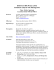### 30 YEARS of MUSICAL MAGIC JOIN US in 2015 for three fantastic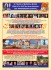### Document 6527965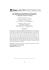### aarrow sign spinners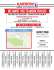### \$7.25 EMPLOYEE RIGHTS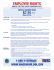### employment application personal employment desired/availability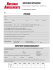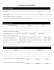### 2011 Texas Lineman`s Rodeo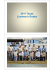### Job Search Form - Service Canada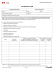### Semiparametric Estimation of a Binary Choice Model with Sample Selection Abstract J¨